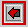# Planetary Calculator### More Planet Variables

 pi ~ 3.141592654 G = gravitational constant c = speed of light Msun = Mass of Sun Rsun = Radius of Sun Lsun = Luminosity of Sun m2AU = meters to AU AU2m = AU to meters s2yr = seconds to years s2hr = seconds to hours r2d = radians to degrees d2r = degrees to radians
 M = mass R = radius rho = density Ve = escape velocity Ps = spin period J2 = oblateness Hr = Hill Radius gs = Surface Gravity

 tilt = tilt a = Semimajor axis i = inclination e = eccentricity Po = orbital period Vo = orbital velocity Rsyn = Synchronous Orbit

Try the Regular Satellite Calculator and the Irregular Satellite Calculator!Return to the Astronomy Workshop

Planetary Calculator written by Amanda L. Proctor and Dr. Douglas P. Hamilton